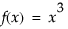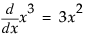Derivative takes the first derivative of an expression with respect to names you specify in the second argument. A single name might be entered as this second argument; or multiple values can be specified in a list, in other words, surrounded by braces.
Note: Derivative is also available as an editing command inside the formula editor (calculator), located on the drop-down list in the top center of the formula editor (above the keypad). To use it, highlight a single variable in the expression (to designate which variable the derivative should be taken with respect to), then select the Derivative command from the menu. The whole formula is replaced by its derivative with respect to the highlighted name.
For, the first derivative is f ’(x) or.
If you want an efficient expression to take the derivative with respect to several variables, then the variables are specified in a list. The result is a list containing a threaded version of the original expression, followed by expressions for the derivatives. The expression is threaded by inserting assignments to temporary variables of expressions that are needed in several places for the derivatives.
NumDeriv takes the first numeric derivative of an operator or function with respect to the value of the first argument by calculating the function at that value and at that value plus a small delta (Δ) and dividing the difference by the delta. NumDeriv2 computes the second numeric derivative in a similar fashion. These are used internally for nonlinear modeling but are not frequently useful in JSL. Note that these functions do not differentiate using a variable, but only with respect to arguments to a function. In order to differentiate with respect to x, you have to make x one of the immediate arguments, not a symbol buried deep into the expression.
Suppose to differentiate = 3x2 at the value of = 3. The incorrect way would be to submit
The correct way is to make x an argument in the function.
 Function Syntax Explanation Derivative Derivative(expr, {name, ...}) Returns the derivative of the expr with respect to name. Note that the second argument can be specified in a list with braces { } or simply as a variable if there is only one. Give two lists of names to take second derivatives. NumDeriv NumDeriv(expr) Returns the first numeric derivative of the expr with respect to the first argument in the expression. NumDeriv2 NumDeriv2(expr) Returns the second numeric derivative of the expr with respect to the first argument in the expression.
Invert Expr(expression, name, y)
 • expression is the expression to be inverted, or the name of a global containing the expression
 • name is the name inside expression to unwind the expression around
 • y is what the expression was originally equal to
y = sqrt(log(x))
y2 = log(x)
exp(y2)=x
Invert Expr supports most basic operations that are invertible, and makes assumptions as necessary, such as assuming you are interested only in the positive roots, and that the trigonometric functions are in invertible areas so that the inverse functions are legal.
F, Beta, Chi-square, t, Gamma, and Weibull distributions are supported for the first arguments in their Distribution and Quantile functions. If it encounters an expression that it cannot convert, Invert Expr() returns Empty().
JSL provides a Simplify Expr command that takes a messy, complex formula and tries to simplify it using various algebraic rules. To use it, submit
Simplify Expr also unwinds nested If expressions. For example:
The Maximize and Minimize functions find the factor values that optimize an expression. The expression is assumed to be a continuous function of the factor values.
result = Maximize(objectiveExpression,{list of factor names}, <<option(value))
result = Minimize(objectiveExpression,{list of factor names}, <<option(value))
objectiveExpression is the expression whose value is to be optimized, and can either be the expression itself, or the name of a global containing a stored expression.
{list of factor names} is an expression yielding a list of names involved in objectiveExpression.
The following example uses Minimize to find the least squares estimates of this exponential model, with data taken from the Nonlinear Example/US Population.jmp sample data table.